# What is A Logic Gate - Beginner's Guide

## 1. What is a Logic Gate?

Logic gates are small digital electronic devices that perform a Boolean function with two inputs and provide an output. The data are the binary ones. Logical 1 is true or high, and logical 0 falls to false or low. Based on the logical gate, the logical operation differs, and the output varies. Each logic gate follows a truth table that gives the possible combinations of input and the respective obtained output.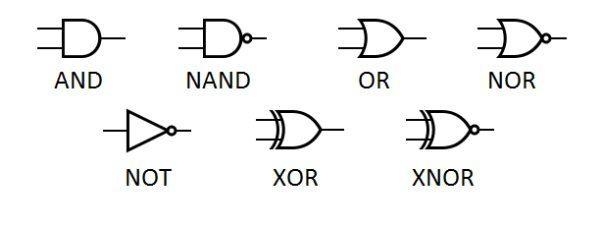The operation of every single logic gate can be easily understood and is similar to addition and multiplication that we already know in ordinary mathematics. A logic gate is identical to a light switch such that it is on when the output is one else off if the production is 0. Various electronic devices are given a form with logic gates, and they get used along with diodes, transistors, and relays. Some of the most widely used transistor families like TTL 7400 series from Texas Instruments and CMOS 4000 series got fabricated with the aid of small-scale logic gates.

## 2. Logic Gate Types and Truth Tables

The underlying symbol representation of the logic gates was depicted in the table format for your easy understanding. There is an article to introduce logic gate symbols.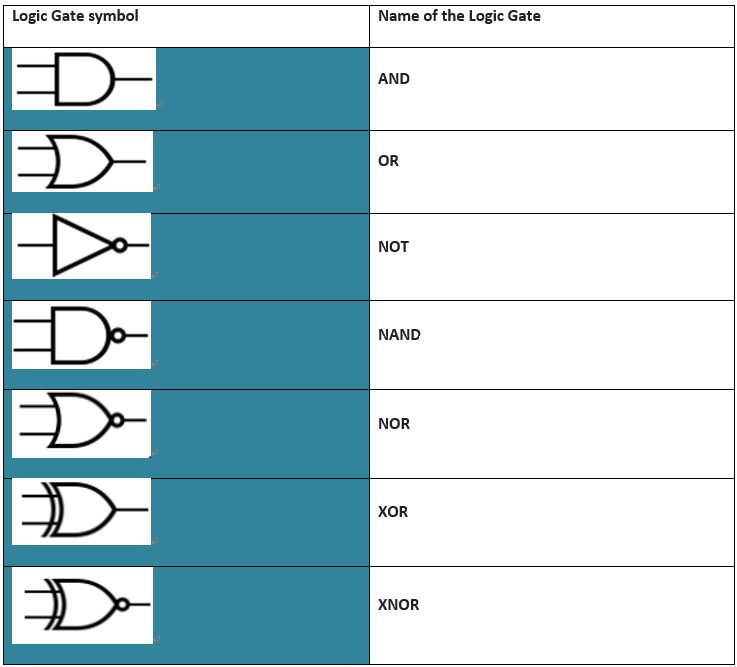In this section, we will discuss in detail the seven basic logic gates and the truth table:

AND Gate

You can list AND gate under the primary logic gate because you can realize some of the upcoming logic gates like NAND from it. It performs multiplication or the Dot (.) operation on the logical inputs. As it can be seen A and B are the two inputs fed to the terminals and O is kept as the output. When you carefully observe the truth table of AND gate, the output is high only when both the inputs are high else in other cases, the output is low.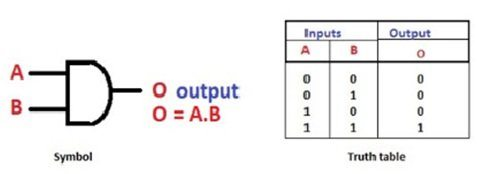Source: www.elprocus.com

OR Gate

OR gate is an essential gate unlike AND as XOR and XNOR can be realized from it. OR gate performs simple addition or ‘+’ operation with the inputs. The output is low or 0 only when both the inputs are 0, and for the rest of the cases, the output is high or logical 1.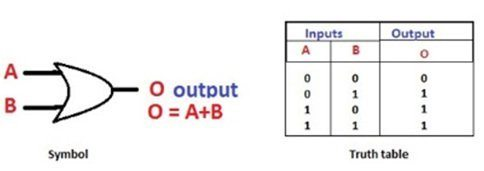Source: www.elprocus.com

NOT Gate

NOT gate is the simplest among all the other logic gates. It performs an inversion operation over the single input. Only one terminal is available in NOT, and if the data is 1, the production is 0, and if the input is 0, the output is 1.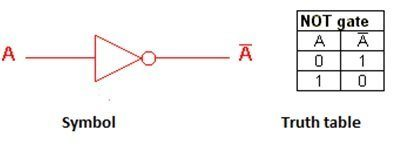Source: www.elprocus.com

NAND Gate

AND gate followed by a NOT gate is the actual concept behind the NAND logic gate, one among the universal gates. When you invert the output of the AND gate, the resultant is the output obtained at the other terminal. Look at the below truth table for further understanding the NAND operation.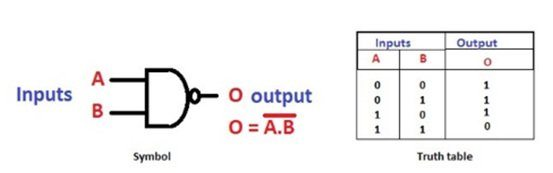Source: www.elprocus.com

NOR Gate

NOR is the combination or Inversion of the logical OR gate and is also a universal logic gate. When the inputs are low or false, the resultant output is high or true.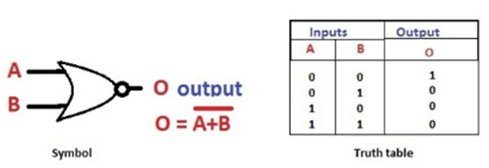Source: www.elprocus.com

XOR Gate

XOR gate is also known as exclusive NOR gate. When you observe the truth table of XOR, you can find that if any input is high, the result is high or true.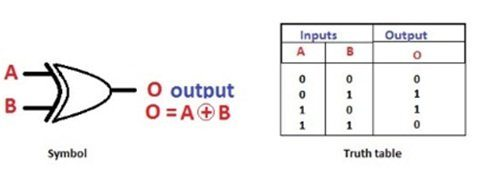Source: www.elprocus.com

XNOR Gate

XNOR or Exclusive NOR gate's basis its operation on the NOR gate. When there is an inversion on the NOR gate, you get the XNOR gate. The output is just opposite to that of the XOR gate. If anyone of the input is high, excluding the condition of both, the output is low or 0.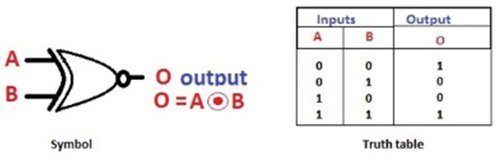Source: www.elprocus.com

## 3. Benefits of Logic Gate Analysis

• Most of the electronic devices or circuits that we use in our routine life constitute logic gates.
• Every digital device of present generation like a laptop, computer, tablet, and mobile phone use logic gates. For instance, let us consider computer memory.
• Logic gate have the functionality of storing the data, and hence they are combined to form a “Latch” circuit and when driven by clock signals, give rise to “Flip-flops.”
• They are known as sequential logic or combinational logic and are responsible for the speed, and complexity.
• An advanced version, Tri-state logic gate finds a place in CPU and buses to perform multiple operations and supports plug-ins too.
• At present, CMOS is the developing technology in microchip development where logic gates are the basic functional blocks.
• Technical microprocessors used in logical circuits consist of more than 100 million gates.

## 4. Logic Gate Use Cases

Electronic Devices: It is to note that every electronic device we use today contains digital circuits and logic gates. Although a logic gate circuit performs basic logical functions, it is still the most crucial part of any digital circuit. The role of logic gates is to make decisions based on digital input. It then simplifies the input by giving one of two outputs, true or false. To simply explain it, a logic gate functions like a light switch.

Industrial Plants: Logic gates are commonly used in industrial plants as safety parameters. We use an OR gate to detect any action in the system. It tells us the occurrence of any unwanted events. That way, Logic gates act as an indicator when the safe value for any breached or exceeded parameters. It gives the output; it indicates that some preventive measures should start working. A simple example is a rise in temperature of a plant reported by logic gates to take action.

Frequency Measurement: We can also use a logic gate to measure the frequency of a wave or pulse. One of the logic gates, the AND gate, is also commonly known as the enable gate. It means that it only allows waves with a specific frequency to pass through and stops the rest. NOT gate is also commonly used as an inventor as it inverts the given input and sends it back as the output.

## 5. Limitations of Logic Gates

Although Logic gates are of high popularity, there are certain limitations:

• For a more complex system or circuit design, logic gate realization isn't possible as it might be confusing to place them correctly and connect them.
• Circuits employing logic gate implementation consume more energy than acceptable.
• Logic circuits require battery power systems or portable power sources.

## 6. History of Logic Gates

Primarily, Gottfried Leibniz refined the idea of a binary system. He also proposed that one can also combine arithmetic and logical principles using the binary number system in 1705. Later in the year 1854, George Boole discovered the concept of Boolean algebra that does the consistent way of comparing numbers to construct decisions. He then published his work in the book titled “An Investigation of the Laws of Thought on Which Are Founded the Mathematical Theories of Logic and Probabilities.” He wished to showcase how mathematical form can represent human reasoning.

Later, in 1886, the concept of logical operation in the electrical switching circuit was described by Charles Peirce. In the meantime, fleming valve got used as logic gates, and relays got replaced by vacuum tubes in 1907. In 1954, Walther Bothe got the Nobel Prize in field of Physics for the invention of the first modern electronic AND gate in the year 1924. Claude Shannon then cited the notion of Boolean algebra in 1937 for the design of switching circuits. Still, research and analysis prevail for the development of molecular logic gates.

## 7. Logic Gates Examples

Full adder is one such good example for the usage of logic gates. Full adder operates on three inputs and yields two outputs, such as Sum and Carry. Widely used for calculation purpose, it performs the addition operation of the given data. This process takes place in seconds as the switching time is fast when compared to that of the analog circuits. Carry-look-ahead adder, BCD adder, and half adder are other categories of adder applications used in digital channels.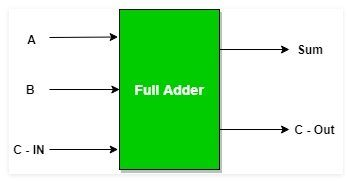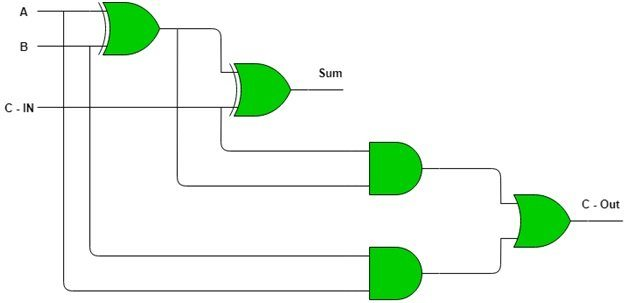Source: www.geeksforgeeks.org

7-Segment Display in a Calculator

Hope you have used calculators and it is our next example that depicts the combinations of logic gates. Although we give our input in the form of numbers, this is what happens within the device. Every segment included in the display is connected to a set of logical gate connection and gets denoted as a, b, c, d, e, f, and, g. For example, when you press 1 on the interface, the below process takes place, and the segment f and e get highlighted or glows on display.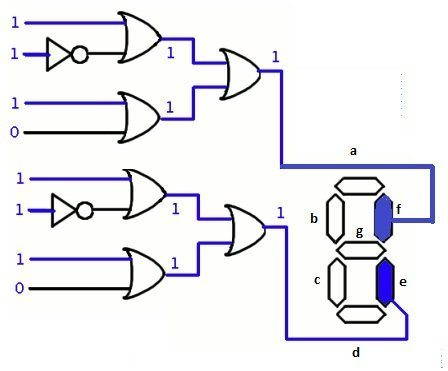Source: www.explainthatstuff.com

## 8. How to Create a Logic Gate with EdrawMax?

Before starting the design process, you should be clear about the circuit requirements, and, plan on how to place them without confusions. You can also have paperwork on the design on how it should be as it will make your process easier in minutes.

Step 2: Once completed, open the software and click on the “Libraries” option from the toolbar.

Step 3: Select the “Circuits and logic diagram” option, and you will see some of the options like “Analog and digital logic,” and “Integrated circuit components.”

Step 4: Click on the “Analog and digital logic” option from the library and start creating your analog circuit with the help of logic gate functions that appear on the left side.

Step 5: After inserting a gate, you can configure it by clicking on the configuration button. You can change the gate type, input, and output.

## 9. Logic Gate FAQ

##### How does a Logic Gate work?

A logic gate deals with the flow of electric current. You have to give input to it, and if the transfer is on, it allows the current to pass through it. The logic gate usually describes the conditions for the current flow you are using as a switch. With Logic gates, you can perform various binary operations such as addition, multiplication, and division.

##### What are the seven Logic Gate?

These are the seven basic logic gates, AND, OR, NOT, NAND, NOR, XNOR, XOR. All of these gates perform different binary operations. Take AND gate as an example. It returns the output as true when all the inputs are true; meanwhile, OR gate returns true either one of the inputs is true.

##### How to change Logic Gate to NAND Gate?

You can use the NAND gate to change any logic gate. If you give NAND gate the same input, then it will act as NOT gate. When there are two inputs for a NAND gate, and you provide the output as input to another NAND gate, it will serve as an AND gate. If you give one input to one NAND gate and another input to another NAND gate and then use both of their outputs as input for a new NAND gate, it will give you the same output as an OR gate.

### Related Articles